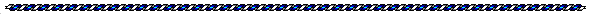The Works of Jerry Iuliano  Ancient Numbers Revealed in Scientific Formulas   Compiled by Joseph E. Mason

New Formula for Electron/Planck Mass Ratio

This is strange. The ratio of the electron mass to the Planck mass is a dimensionless entity because kilograms cancel:

 Me = electron mass = 9.109383761 * ( 10 ^ - 31 ) kg   Mp = Planck mass = 2.176449594 * ( 10 ^ - 8 ) kg

 Me / Mp = 4.185432912 * ( 10 ^ - 23 )

. . . the kilogram dimension cancels because . . . kg/kg = unity. However, due to the recently discovered formula:

 16 * ( Pi^2 ) * E * ev * ( 10 ^ 6 ) / h / ( c^4 ) = Me / Mp

. . . a new form of dimensioning occurs:

 E = permitivitty of space = 8.854187818 * ( 10 ^ - 12 )F/m  ev = elementary charge of electron = 1.602176532 * ( 10 ^ - 19 ) C   h = Plancks constant = 6.6260693 * ( 10 ^ - 34 ) J c = speed of light = 299792458 m/s

. . . the dimensioning can be shown as:

 farad * coulomb * second per meter per joule per second per meter   F * C * s / m / J / s / m

. . . which cancels to:

 farad * coulomb per joule per meter squared   F * C / J / (m ^ 2 )

. . . according to SI derived units:

 F = capacitance = ( s ^ 4 ) * ( A ^ 2 ) / ( m ^ 2 ) / kg   C = coulomb = s * A ......( A = amperes ) J = joule = ( m ^ 2 ) / kg / ( s ^ 2 ) m = meter s = second kg = kilogram

. . . setting into the new form:

 ( s ^ 4 ) * ( A ^ 2 ) / ( m ^ 2 ) / kg * s * A / ( m ^ 2 ) / kg * ( s ^ 2 ) / ( m ^ 2 )

. . . which reduces to:

 ( s ^ 6 ) * ( A ^ 3 ) / ( m ^ 6 ) / ( kg ^ 2 ) = unity

. . . according to the new discovery , the above dimensioning cancels to unity!!

seconds to the sixth power times amperes cubed divided by meters to the sixth power divided by kilograms squared equals unity!!

. . . the permitivitty of space . . . E . . . has dimensionals:

 farad per meter = ( s ^ 4 ) * ( A ^ 2 ) / ( m ^ 3 ) / kg

. . . when reduced from the unity equation shows the permitivitty of space dimensioning with a residue dimensioning of:

 ( s ^ 2 ) * A / ( m ^ 3 ) / kg

. . . out of this residue dimensionals is the inverse of the magnetic flux in webers:

 magnetic flux = volt * second = ( m ^ 2 ) * kg / ( s ^ 2 ) / A   1 / v / s = ( s ^ 2 ) * A / ( m ^ 2 ) / kg

. . . so the new formula has a dimensioning parameter of the permitivitty of space divided by the magnetic flux with a single dimensional of length . . . meter . . . as residue. The only formula that I know of that uses meter cubed is the gravitational constant Gn !!:

 Gn = 6.6742 * ( 10 ^ - 11 ) ( m ^ 3 ) / kg / ( s ^2 )

. . . conclusion is that the electron/Planck mass ratio is a result of the permitivitty of space having a built in magnetic flux determined by a gravitational like tensor in three dimensional space... all canceling to unity!!!

J. Iuliano
(Sent via e-mail February 5, 2006)Definitions

Me = electron mass = 9.1093826 * ( 10 ^ - 11 ) grams
Gn = gravitational constant in newtons = 6.6742 * ( 10 ^ - 11 ) k^3/m/s
c = speed of light = 299792458 meters/seconds
h = Planck's constant = 6.62606891 * ( 10 ^ - 34 ) Joules
E = permittivity of space = 8.854187818 * ( 10 ^ - 12 ) Fm<
Amperes = the rate at which electricity flows through a conductor

_________________________________

Help Pages